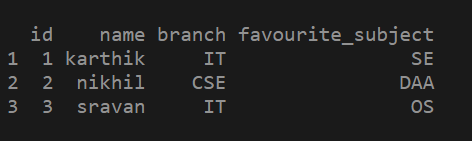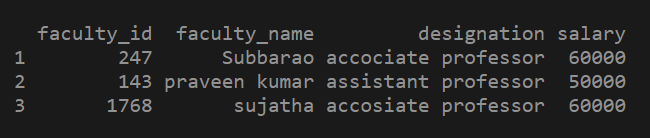# How to create a DataFrame from given vectors in R ?

In this article we will see how to create a Dataframe from four given vectors in R. To create a data frame in R using the vector, we must first have a series of vectors containing data. The data.frame() function is used to create a data frame from vector in R.

Syntax:

`data.frame(vectors)`

Example 1. Creating dataframe from given 4 vectors.

## R

 `# creating a vector with some value ` `id = ``c``(1, 2, 3)`   `# creating another vector with some value` `name = ``c``(``"karthik"` `, ``"nikhil"` `, ``"sravan"``) `   `# creating another vector with some value` `branch = ``c``(``"IT"` `, ``"CSE"` `, ``"IT"``) `   `# creating another vector with some value.` `favourite_subject = ``c``(``"SE"` `,``"DAA"` `, ``"OS"``) `   `# passing the vectors into data.frame() function ` `# as parameters` `df1=``data.frame``(id, name, branch, favourite_subject) `   `# printing the data frame.` `print``(df1) `

Output:Example 2:

## R

 `# creating a vector 1 with some values` `faculty_id = ``c``(247, 143, 01768) `   `# creating a vector 2 with some values` `faculty_name=``c``(``"Subbarao"``, ``"praveen kumar"``, ``"sujatha"``)`   `# creating vector 3 with some values` `designation=``c``(``"accociate professor"``, ``"assistant professor"``,` `              ``"accosiate professor"``)`   `# creating vector 4 with some data ` `salary = ``c``(60000, 50000, 60000)`   `# passing the vectors to the data.frame() function` `df3 = ``data.frame``(faculty_id, faculty_name, designation,salary) `   `# printing the data frame created with 4 vectors` `print``(df3)`

Output:Whether you're preparing for your first job interview or aiming to upskill in this ever-evolving tech landscape, GeeksforGeeks Courses are your key to success. We provide top-quality content at affordable prices, all geared towards accelerating your growth in a time-bound manner. Join the millions we've already empowered, and we're here to do the same for you. Don't miss out - check it out now!

Previous
Next